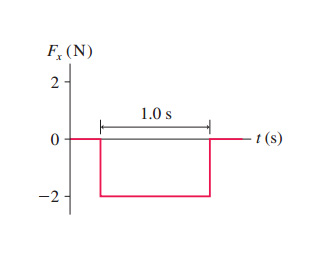# Problem: A 2.0 kg object is moving to the right with a speed of 1.0 m/s when it experiences the force shown in the figure. What are the object’s speed and direction after the force ends?

###### FREE Expert Solution

For an F-t graph, the area under the curve gives the change in momentum.

The change in momentum is referred to as impulse.

$\begin{array}{rcl}\mathbf{∆}\mathbf{P}& \mathbf{=}& {\mathbf{F}}_{\mathbf{x}}\mathbf{∆}\mathbf{t}\\ \mathbf{m}{\mathbf{v}}_{\mathbf{f}}\mathbf{-}\mathbf{m}{\mathbf{v}}_{\mathbf{0}}& \mathbf{=}& {\mathbf{F}}_{\mathbf{x}}\mathbf{∆}\mathbf{t}\end{array}$

80% (454 ratings)###### Problem Details

A 2.0 kg object is moving to the right with a speed of 1.0 m/s when it experiences the force shown in the figure.What are the object’s speed and direction after the force ends?

Frequently Asked Questions

What scientific concept do you need to know in order to solve this problem?

Our tutors have indicated that to solve this problem you will need to apply the Impulse with Variable Forces concept. You can view video lessons to learn Impulse with Variable Forces. Or if you need more Impulse with Variable Forces practice, you can also practice Impulse with Variable Forces practice problems.